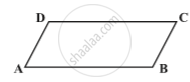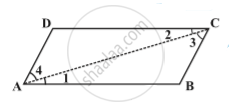Share

# Kinds of Quadrilaterals - Elements of a Parallelogram

#### notes

There are four sides and four angles in a parallelogram. Some of these are equal. There are some terms associated with these elements that you need to remember.
Given a parallelogram ABCD in following fig.bar (AB) and bar (DC), are opposite sides. bar (AD) and bar (BC) form another pair of opposite sides.
∠A and ∠C are a pair of opposite angles; another pair of opposite angles would be ∠B and ∠D.
bar (AB) and bar (BC) are adjacent sides. This means, one of the sides starts where the other ends.
∠A and ∠B are adjacent angles. They are at the ends of the same side. ∠B and ∠C are also adjacent.

Property: The opposite sides of a parallelogram are of equal length.
Given : A parallelogram ABCD , AB || CD and AD || BCProve : AB = CD and AD = BC
Draw any one diagonal AC.
Proof : In ∆ ABC and  ∆ CDA , we have :
∠1 = ∠2   ...  [Alternate angles, AB ||CD and CA is transversal ]
∠3 = ∠4   ...  [Alternate angles AD || BC and AC is transversal ]
∆ ABC ≅ ∆ CDA   ...[ASA  Axioms]
AB = CD and AD = BC  [C.P.C.T]

### Shaalaa.com

Elements of a parallelogram [00:08:01]
S
0%

S